Materials Science and Technology 21 (2005) 1059-1062

Kinetics of the crystallization of Ni–Zn ferrite powders prepared by the hydrothermal method

R. L. Mancera,* G. Ridlová, Y. Wang & H. K. D. H. Bhadeshia

Department of Materials Science and Metallurgy,

University of Cambridge, Pembroke Street, Cambridge CB2 3QZ, United Kingdom

*Western Australian Biomedical Research Institute, GPO Box U1987, Perth WA 6845, Australia.

Abstract

Recently published data on the precipitation of Ni-Zn ferrite powders prepared by the hydrothermal method have been analysed in a manner consistent with Avrami theory.  The results indicate that the value of the time exponent is due to the growth of spherical particles in a solution which becomes progressively depleted of reactants.  The analysis also indicates that the process can be considered to be one in which the particles all start growth from the initiation of the reaction.

Keywords:  ferrite, crystallization kinetics, Avrami theory, hydrothermal method

1. Introduction

Ferrites are a group of crystalline ceramic materials composed of iron oxide in combination with some other metal(s).  They are used in magnetic, electronic and microwave devices due to their high magnetic permeability .

Spinel-type ferrite powders can be prepared by a variety of methods [2-7], including the hydrothermal method, which utilizes aqueous solvents under pressure and at temperatures above the normal boiling point of water in order to speed up the reaction between solids [8,9].  The basic mechanism for the hydrothermal formation of ceramic oxide particles involves a dissolution/precipitation mechanism.  As the precursors (such as hydroxides) of component oxides are heated, their solubility increases.  Eventually, a sufficient concentration of the components exists in solution to initiate formation of the more stable oxide phase.  This process follows the general scheme of Schikorr’s reaction .  The driving force in these reactions is the difference in solubility of the oxide phase from the least soluble precursor or intermediate.  Reaction parameters such as the solution pH, temperature and hydrothermal duration determine the nature of the final products [5-9,11,12].

Recently Wang and Kung reported the use of the hydrothermal method to promote the crystallization of nanosized powders of Ni0.5Zn0.5Fe2O4 spinel ferrites .  The crystallization kinetics in the temperature range 100 °C to 150 °C for different durations was investigated by X-ray diffraction (XRD).  Using an empirical application of Avrami theory, they reached the conclusion that the time-dependence of precipitation can be explained in terms of nucleation alone.  A crystallization activation energy of 56.2 kJ/mole was reported. Unfortunately, there are inconsistencies in their analysis and interpretations, as described below.  The purpose of the present work was to deal with these difficulties and reach conclusions which are based on the mechanism of precipitation.  We begin with a brief explanation of Avrami theory in order to set the scene.

2. Classical overall transformation kinetics theory

The evolution of volume fraction during a solid-state transformation is usually described using the classical Kolmogorov-Johnson-Mehl-Avrami theory [14-18].  This theory makes use of the concept of extended volume to estimate the real volume fraction of the phase transformation product when hard impingement between growing particles is taken into account.  If unrestricted growth in the extended space is assumed, some particles will grow in a region that has already been transformed.  However only those particles that lie in a previously untransformed region of the matrix can contribute to a change in the real volume of the product phase (α):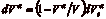(1)

where V is the total volume, Vα is the volume of α and Veα is its corresponding extended volume.

If we consider a simple case where the product phase grows isotropically at a constant rate G and with a nucleation rate per unit volume IV, then the volume vτ of a particle nucleated at time t = τ is given by: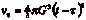(2)

We can then write the change in extended volume over the time interval τ and τ + as: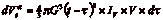(3)

If we then substitute the above into eq. (1), integrate over time and rearrange we obtain the following expression: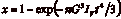(4)

where x = Vα /V is the volume fraction of the product phase.  The above derivation has been made with the assumptions of random nucleation, a constant nucleation rate and a constant growth rate.  Since there are different possibilities we can express eq. (4) in a more general form as: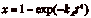(5)

where kA and n are constants characterising the reaction as a function of time, temperature and other variables.  The values of kA and n can be obtained from experimental data by plotting ln [-ln(1-x)] versus ln (t).  The specific values of kA and n depend on the nature of nucleation and growth.  For example, a constant nucleation and growth rate leads to a time exponent n = 4, while an exponent of n = 3 is obtained if it is assumed that no nucleation is needed but the growth rate is constant .

3. Determination of the kinetics of crystallization of nanosized Ni-Zn ferrite powders

Wang and Kung used the hydrothermal method to produce ultrafine powders (between 10 and 20 nm in size) of Ni0.5Zn0.5Fe2O4 from solution nitrate precursors and used aqueous ammonia to obtain the desired pH.  The authors investigated the crystallization of these powders at different pH, temperatures between 100 °C and 180°C and process durations of up to 8 hours .  It was found that a single, well-developed, spinel phase was obtained at an optimum pH of 9.0, which was subsequently used in all other experimental determinations.

The XRD integral intensity of the (311) peak of the spinel phase was used to investigate the crystallization kinetics at 100 °C, 110 °C, 120 °C, 130 °C and 150 °C for process durations of up to 8 hours.  Wang and Kang used the XRD intensity obtained at the longest duration (8h) and the highest temperature (180°C) to represent the maximum reaction expected; the fractions for other conditions were therefore derived by dividing the integrated intensity by that for the 180°C, 8h experiment.  Henceforth all fractions (x) refer to the fraction normalised against the 180°C, 8h reference.

The general form of the empirical Avrami transformation kinetic equation (eq. (5)) was used to evaluate the XRD data.  By taking logarithms and rearranging eq. (5), it can be re-written in a linear form: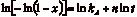(6)

where a plot of ln [-ln(1-x)] as a function of ln t yields the values of n and kA.

We have extracted the isothermal crystallization curves directly from Fig. 4 of reference  .  The data are shown in Table 1 and Figure 1.

Fig. 1 shows that the maximum normalised fraction x of crystals at 150 °C is 0.7.  This clearly indicates that at 150 °C and after 8 h the crystallization process has not reached a 100% transformation when compared against the reference (180°C, 8h, ).   Furthermore, the levelling off of the curves at the lower temperatures of 120, 130 and 150 °C shown in Fig. 1, is rather odd given that the value of x is only about 0.5. This suggests that the composition (of precursors) in the matrix is in fact changing with time, which was not considered in the original analysis .

Fig. 2 shows the Avrami plots for each of the temperatures investigated by considering eq. (6).  The reported values of the n constant of 0.88 at 100 °C, 1.15 at 110 °C, 0.82 at 120 °C, 0.86 at 130 °C, and 1.218 at 150 °C, giving a mean value of n = 0.99 .  From this result it was inferred that the only contribution to the time exponent in the Avrami equation is from nucleation. This is unphysical since growth obviously occurs and must contribute to the time exponent.  However, it is evident that the non-linear plots shown in Fig. 2 that the data do not fit the Avrami equation.   Linear regressions on the data revealed rather different values of n from those reported :  0.86 at 100°C, 0.84 at 110 °C, 0.50 at 120 °C, 0.39 at 130 °C, and 0.39 at 150 °C.  Our analysis revealed that this is because the original analysis seems to have used only the initial linear part of the plots, i.e. only the first three datapoints (only two at 150°C) were used to determine n.  It also was found that in the derivation of the activation energy the ln kA value at the highest temperature (150 °C or 423 K) was omitted .

4. Re-evaluation of the experimental data

It is clear from the data that the precipitation reactions never go to completion, undoubtedly because the concentration of reactants in solution changes during the course of the reactions.  As recent thermodynamic calculations [19,20] indicate, the maximum extent of reaction is a function of temperature, with solubility decreasing as the temperature increases.  It follows that the crystallized fraction x must be normalized using the maximum fraction possible at any temperature .  Therefore, the observed crystallized fraction x was divided by the maximum crystallized fraction observed after 8 h at each individual temperature (the data indicate that this is sufficient time to reach stasis, at least for the 120, 130 and 150 °C cases), as shown in Table 2 and Fig. 3.

Fig. 4 shows the corresponding Avrami plots for each of the temperatures investigated by considering eq. (6), which on the whole show the expected linear fit to the Avrami equation.  The n values obtained are:  1.43 at 100°C, 1.49 at 110 °C, 1.72 at 120 °C, 1.25 at 130 °C, and 0.83 at 150 °C, giving a mean value of n = 1.34 ± 0.05. The data for 150°C are the most approximate given that there is a clear and unexplained change in slope as the reaction proceeds.

The growth of the ferrite powders is controlled by the transport of solute, a process which must slow down as the reaction proceeds and the reactants are depleted.  In the absence of soft-impingement, each particle will increase its size q in proportion to t1/2 .  The precipitate volume is proportional to q3 for spherical particles and hence is proportional to t3/2.  The actual time exponent may be somewhat smaller than 1.5, particularly at the later stages of precipitation, because the solute fields of different particles interact (i.e., soft impingement occurs).  Our reanalysis indicates that n = 1.34, which is consistent with this growth mechanism, assuming that particles start growth at time zero.

This interpretation indicates that the barrier to nucleation is negligible, which is possible if the initial mixture is impure.  An examination of transmission electron micrographs reveals that the ferrite powders while not exactly spherical or uniform in shape , have morphologies consistent three-dimensional precipitate growth as assumed in eq. (2).  More importantly, the micrographs also reveal that the growing particles are uniform in size, consistent with the conclusion that they all start growing from time zero.

The corresponding values of ln kA for each temperature are listed in Table 3, giving an activation energy EA = 57.4 ± 14.4 kJ/mole from the corresponding Arrhenius plot.  This is essentially the same as that reported in , but the agreement is fortuitous since their reported value would have been 45.5 kJ/mole had they used all the data.

Our activation energy is significantly lower than the value of 329-353 kJ/mole reported for the solid state reaction formation of Ni-Zn-ferrites .  However, hydrothermal synthesis involves precipitation in a fluid, so it is not surprising that the activation energy is lower by an order of magnitude, and we speculate that it refers to the attachment of molecules to the growing particles.

5.  Conclusions

Published data  on the precipitation of Ni-Zn ferrite powders prepared by the hydrothermal method have been analysed in a manner consistent with Avrami theory.  The results indicate that the value of the time exponent is consistent with the growth of spherical particles in a solution which becomes progressively depleted of reactants.  The analysis also indicates that the process can be considered to be one in which the particles all start growth from the initiation of the reaction.

References

 A. Goldman, Handbook of Modern Ferromagnetic Materials,
Kluwer, 1999.

  K. Tkácová, V. Sepelák, N. Stevulová, J. Solid State Chem. 123 (1996) 100.

  P.A. Lane, P.J. Wright, M.J. Crosbie, A.D. Pitt, C.L. Reeves, B. Cockayne, A.C. Jones, T.J. Leedham, J. Crystal Growth 192 (1998) 423.

  Z. Youcai, R. Stanforth, Miner. Eng. 13 (2000) 1417.

  J.H. Lee, C.K. Kim, S. Katoh, R. Murakami, J. Alloys Compounds 325 (2001) 276.

  W.J. Dawson, J. Am. Ceram. Soc. 67 (1988) 1673.

  P. S. Anil Kumar, J. J. Shrotri, C. E. Deshpande, S. K. Date, J. Appl. Phys. 81 (1997) 4788.

  S. Komarneni, E. Fregeau, E. Breval, R. Roy, J. Am. Ceram. Soc. 71 (1988) 26.

  C.K. Kim, J.H. Lee, S. Katoh, R. Murakami, M. Yoshimura, Mater. Res. Bull. 36 (2001) 2241.

  G. Schikorr, Z. allg. Chem. 212 (1938) 33.

  K. Byrappa, M. Yoshimura, Handbook of Hydrothermal Technology, Noyes 2001.

  K. Byrappa, Hydrothermal Growth of Crystals, Pergamon Press, Oxford, 1991.

  H.-W. Wang, S.-Ch. Kung, J. Magn. Magn. Mater. 270 (2004) 230.

  M. Avrami, J. Chem. Phys. 7 (1939) 1103.

  W.A. Johnson, R.F. Mehl, Trans. AIME 135 (1939) 416.

  M. Avrami, J. Chem. Phys. 8 (1940) 212.

  M. Avrami, J. Chem. Phys. 9 (1941) 177.

  J.W. Christian, Theory of Transformations in Metals and Alloys, Pergamon Press, Oxford, 1975.

  L. Xiang, Y.P. Yin, Y. Jin, J. Mater. Sci. 37 (2002) 349.

  T. Adschiri, Y. Hakuta, K. Sue, K. Arai, J. Nanoparticle Res. 3 (2001) 227.

  L.A. Bashkirov, M.G. Bashkirova, Vses. Soveshch., 1968, p. 216.

Figure Captions

Fig. 1.  Isothermal crystallization curves at (a) 100 °C, (b) 110 °C, (c) 120 °C, (d) 130 °C, and (e) 150 °C .

Fig. 2.  Avrami plots of ln [-ln(1-x)] against ln t for isothermal crystallization.

Fig. 3.  Normalized crystallization curves at (a) 100 °C, (b) 110 °C, (c) 120 °C, (d) 130 °C, and (e) 150 °C.

Fig. 4.  Avrami plots of ln [-ln(1-x)] against ln t for the normalized crystallization data.

Figure 1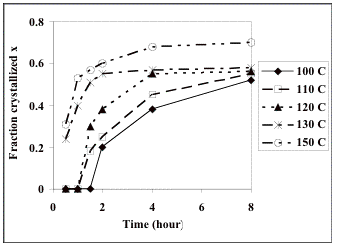Figure 2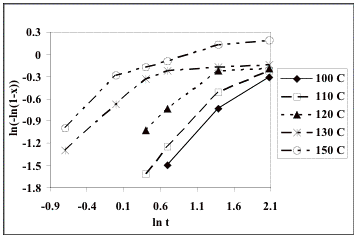Figure 3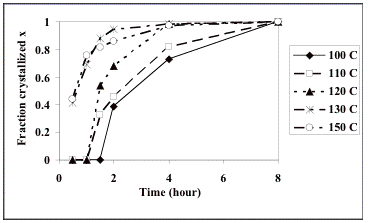Figure 4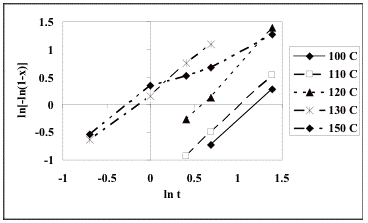Table 1.  Isothermal crystallization data for the formation of Ni-Zn ultrafine powders.

 Time (hour) Fraction crystallized x 100 °C 110 °C 120 °C 130 °C 150 °C 0.5 0 0 0 0.24 0.31 1 0 0 0 0.4 0.53 1.5 0 0.18 0.3 0.51 0.57 2 0.2 0.25 0.38 0.55 0.6 4 0.38 0.45 0.55 0.57 0.68 8 0.52 0.55 0.56 0.58 0.7

Table 2.  Normalized crystallization data

 Time (hour) Fraction crystallized x 100 °C 110 °C 120 °C 130 °C 150 °C 0.5 0 0 0 0.41 0.44 1 0 0 0 0.69 0.76 1.5 0 0.33 0.535714 0.88 0.8 2 0.39 0.46 0.678571 0.95 0.86 4 0.73 0.82 0.982143 0.98 0.97 8 1 1 1 1 1

Table 3.  Values of ln kA for each crystallization temperature from the normalized data

 T (K) 373 383 393 403 423 ln kA -1.717 -1.53 -1.003 0.215 0.157